# Algorithm Complexity Simplified

Algorithm Complexity is the favorite interview topic of FAANG companies and vital for choosing tons of available solutions for a single problem. If you go by textbook definitions and concepts, it will leave you scratching your head in most cases. This blog post will put the Algorithm Complexity in its simplest form, required to meet the purpose of real-life coding challenges and interviews.
Disclaimer:

• The concepts explained here might not help you crack competitive examinations like GATE as they focus on mathematical definition rather than practical usage. But this will simplify things to make those concepts understand easily.
• The pre-requisite to this blog is an understanding of various sorting algorithms, arrays, data structures (stack, queue, binary tree), and recursion.

# Why Algorithm Complexity?

To understand the need for Algorithm Complexity, let’s go for purchasing a Car. To simplify things, let’s assume we’ve already fixed a budget and filtered two cars. Both the vehicles share the same feature set and price; which one you’ll pick? In most cases, you’ll go for the most fuel-efficient car, which is one of the performance criteria. Similarly, Algorithm Complexity represents an algorithm’s performance criteria and helps us pick from various available solutions.

# What is Algorithm Complexity?

Algorithm Complexity essentially represents the rate of increase in time (taken by the algorithm) as the input size increases. For example, how much time a web page takes to load when 5 users are using it versus 5 Million at the same time? And yes, this is relatable to functions we all learned in school, also known as Asymptotic Functions (see this article for a refresher). Therefore, Algorithm Complexity is represented in Asymptotic Annotations; Big Omega, Big O, and Big Theta.
Note: There are 2 complexities for an algorithm, one is Time, and another is Space. We’re not going to talk about Space complexity as it’s no longer an issue with a wide availability of high memory hardware (hosts) cheaply. It used to be a constraint when memories were still in MBs, and we were still using floppies.

# Big Omega, Big O, and Big Theta

Big O (or simply ‘O’) describes an upper bound on time. Meaning, the algorithm will not take more than Big O time in any case. It can take less time than Big O (on specific input values) but not more than Big O in any case. For example, searching number 5 in the array {1, 2, 3, 4, 5} will take 5 iterations, while searching number 1 will take 1 iteration. Since Big O describes the upper bound, the time complexity for searching a number in an array of size N is O(N).

Big Omega (or simply ‘Ω’) describes a lower bound on time. Meaning, the algorithm will not take less than Big Ω time in any case. It can take more time than Big Ω (on specific input values) but not less than Big Ω. In the array example above, the Big Ω complexity is Ω(1) as searching for the number won’t take less than 1 iterations.

Big Theta (or simply ‘Θ’) describes tight bound on time. Meaning, the algorithm will take exactly Big Θ time, not less or more (+/- a constant time). For example, a web page will be displayed within 5 milliseconds if the number of users is between 50–60. In this case, the complexity will be Θ(55) with +/- 5 users.

Moving forward, the algorithm complexity will be represented only in Big O as the industry doesn’t care about Big Omega or Big Theta. But why? from the practical point of view, both Big Omega and Big Theta don’t provide any value. For example, the website will display the web page in 1 millisecond at best, or the website will display the web page in 5 milliseconds if the number of users is between 50–60. Both of these are exceptional cases, and what we care about is knowing practical scenarios (such as time taken as the traffic increases).

# Drop the Constant and Non-Dominant terms

Let’s take the below code as an example and determine its complexity.

Every time the function calculateSumAndProduct executes, lines #2, #3, #9, and #10 will run exactly one time, while lines #5 and #6 will run N times (size of the array “nums”). Based on it, the complexity will be O(2N + 4). But as we discussed earlier, the goal of complexity is to determine the rate of increase; we’re not gaining much from being accurate, as seen from the rate of increase graphs below for O(N) and O(2N + 4). And hence, in Big O, constant terms are dropped, making the complexity of the above code O(N).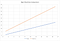Runtime Comparison of O(N) and O(2N+1)

Similarly, the complexity of the non-dominant term is dropped. For example, O(N² + N) will become O(N²).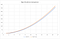Runtime Comparison of O(N²) and O(N²+N)

While we drop Constant and Non-Dominant Terms, remember, the complexity cannot be reduced further if statements are getting executed a different number of times. For example, the below code’s complexity will be O(N + M) as we cannot be sure what will be the value of N and M (size of num1 and num2 respectively). It’s as good as comparing mangoes with oranges.

# Best, Worst, and Expected Case Complexity

The Big O runtimes are categorized as Best, Worst, and Expected case. Let’s understand this with an example of searching a number in an array.

1. Best Case: When the number we are searching for is found at the first index of the array, the runtime becomes O(1).
2. Worst Case: When the number we are searching is at the last index of the array, the runtime becomes O(N).
3. Expected (Average) Case: In real life, we never get too lucky or unlucky in most cases, and hence Expected Case is the most practical one. The runtime for searching a number in an array is O(N/2) = O(N).

In most cases, the worst and expected case complexities are the same (as seen in the above example as well).

# Runtimes

## Constant Runtime

An algorithm is said to be constant time if it has a fixed set of instructions irrespective of the input. For example, see the code block below

The complexity of the code is O(2) as there are two instructions. Since we drop the constant terms, such complexity is represented as O(1), which is also the best possible runtime complexity for an algorithm. More such examples are accessing the array elements, find if a number is even or odd, find a value on a Map, etc.

## O(N) Runtime

When an algorithm has an N number of instructions, usually in a loop, for example, the below code will execute exactly N instructions depending on the array’s size.

## O(N²) or O(N^N)

When an algorithm has a piece of code with nested blocks, usually nesting of loops, for example, the below code, will execute O(N²) times.

And similarly, if there are 3 nested loops, the complexity is O(N³) and so on…

## O(log N) and O(N log N)

The Divide and Conquer algorithms usually have logarithmic runtimes. For example, searching a number in a sorted array using the Binary Search algorithm has O(log N) runtime. The array’s size is divided in half until the number is found, or the input cannot be divided further.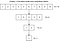Binary Search INPUT on every iteration

Suppose we need to search N numbers from the given sorted array using Binary Search. In that case, the complexity will be O(N log N) as we’ll be dividing the input in half for every number N. Typically, Divide and Conquer sorting algorithms, such as Quick Sort, Merge Sort, etc., are O(N log N) runtime algorithms, which is also the best runtime for a sorting algorithm.

## O(2^N)

These are also known as recursive (see Appendix 1 for more details) runtimes where the code is executed 2^N times for every input N. For example, finding Nth Fibonacci number.

The program will be executed as follows.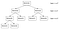Fibonacci function calls after every execution

The complexity of the code is O(2^(N-1)) = O(2^N). These complexities can be generalized as O(branches^depth)

# Cheatsheet for Runtimes Comparison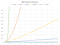Comparison of all runtimes

O(1) < O(log N) < O(N) < O(N log N) < O(N²) < O(2^N)

# Appendix 1: Recursion

A function that calls itself is known as a recursive function. The problems that are too complex for an iterative approach are broken down into smaller ones through recursion — for example, iterating through a Binary Tree. It requires maintaining previous states via Stack/Queue in an iterative approach, while the recursive approach leverages the platform’s stack approach and keeps the code clean.

Note: Recursion is a complex topic in itself, and it’s not possible to cover it in a paragraph. I’ll be covering this topic in detail in another blog.